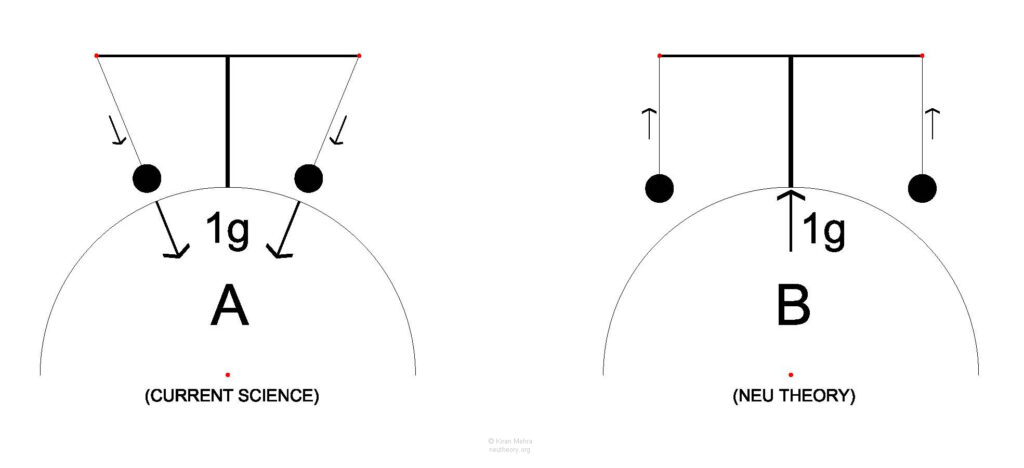NEU Theory

## NEU Theory

The Nature of Physical Reality

## The Direction of Gravity

Our second tool is understanding the direction of a natural force.

We ask the question, what is the direction of the Earth’s gravitational force that we all experience? Since Newton, gravity has been considered a pull, a universal attractive force between matter.

Neu Theory proposes a thought experiment to test this undisputed hypothesis.

See Figure 0.7 – Direction of force – the Cantilevered Pendulums Experiment.

The diagram shows two half circles, representing the Earth.Figure 0.7 – Direction of Force – The Cantilevered Pendulums Experiment

Imagine the Earth’s surface to be a perfect sphere, no atmosphere. Each hemisphere has two giant cantilevered pendulums on a column with stationary bobs subject to Earth’s gravitational force. In A the bobs point to the center of the earth and in B they are parallel to the column.

Question: Which will happen A or B?

This thought experiment may be viable as a real experiment in a scaled down version:

1. Use photons to measure the gap between the strings top and bottom. A smaller gap (A) implies a pull, the same size gap (B) implies a push.
2. Use photons emitted from the cantilevered ball reflecting off a liquid mercury or other reflective surface that follows the curvature of the earth. If the angle of incidence and the angle of reflection are the same gravity is a pull. If the angle of incidence and the angle of reflection are different gravity is a push.

The Neu Theory model uses diagram B in its description of the direction of the Earth’s gravitational force that we all experience, and designates it as one unit of g-rise, a push not a pull. All objects have a g-rise directly proportional to their mass and inversely proportional to their volume.

Neu Theory G-rise is equivalent to the conventional Newtonian gravitational attraction force.

Nothing changes in a practical sense. All formulas will work the same giving the same results, only our perception of what is occurring changes.